### 你GET到了吗？要获得优秀的模型，首先需要清洗数据

• 发布时间：2020-07-08
• 来源：
• 浏览次数：1267

「数据清洗」光定义就这么长，执行过程肯定既枯燥又耗时。# import packagesimport pandas as pdimport numpy as npimport seaborn as sns
import matplotlib.pyplot as pltimport matplotlib.mlab as mlabimport matplotlibplt.style.use('ggplot')from matplotlib.pyplot import figure
%matplotlib inlinematplotlib.rcParams['figure.figsize'] = (12,8)
pd.options.mode.chained_assignment = None
# shape and data types of the dataprint(df.shape)print(df.dtypes)
# select numeric columnsdf_numeric = df.select_dtypes(include=[np.number])numeric_cols = df_numeric.columns.valuesprint(numeric_cols)
# select non numeric columnsdf_non_numeric = df.select_dtypes(exclude=[np.number])non_numeric_cols = df_non_numeric.columns.valuesprint(non_numeric_cols)

cols = df.columns[:30] # first 30 columnscolours = ['#000099', '#ffff00'] # specify the colours - yellow is missing. blue is not missing.sns.heatmap(df[cols].isnull(), cmap=sns.color_palette(colours))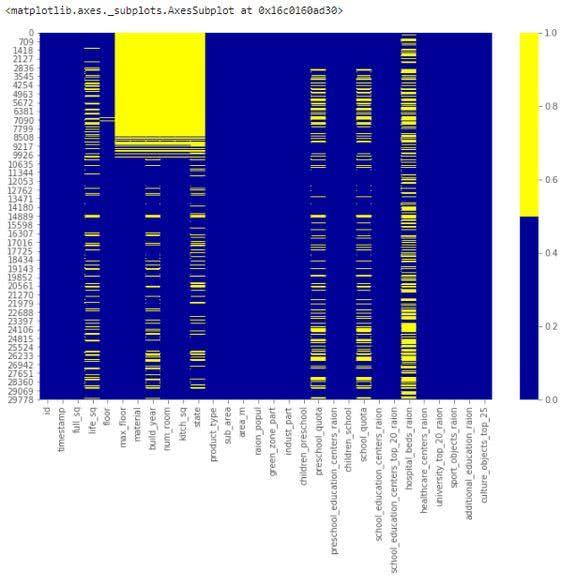# if it's a larger dataset and the visualization takes too long can do this.# % of missing.for col in df.columns: pct_missing = np.mean(df[col].isnull()) print('{} - {}%'.format(col, round(pct_missing*100)))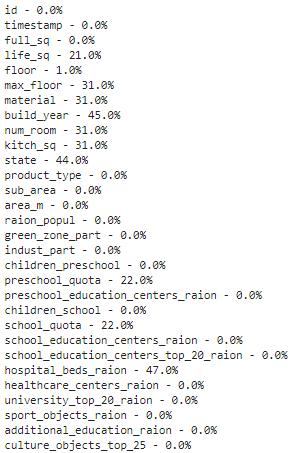# first create missing indicator for features with missing datafor col in df.columns: missing = df[col].isnull() num_missing = np.sum(missing)
if num_missing > 0: print('created missing indicator for: {}'.format(col)) df['{}_ismissing'.format(col)] = missing
# then based on the indicator, plot the histogram of missing valuesismissing_cols = [col for col in df.columns if 'ismissing' in col]df['num_missing'] = df[ismissing_cols].sum(axis=1)
df['num_missing'].value_counts().reset_index().sort_values(by='index').plot.bar(x='index', y='num_missing')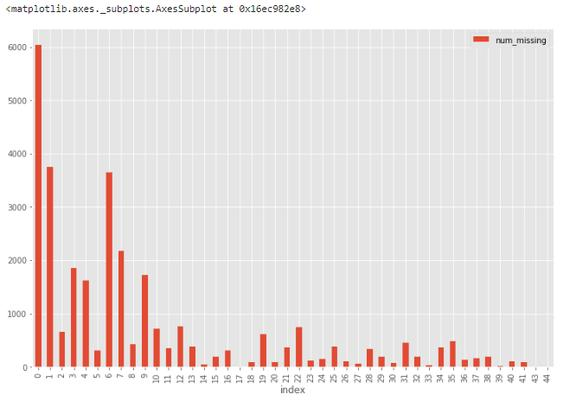# drop rows with a lot of missing values.ind_missing = df[df['num_missing'] > 35].indexdf_less_missing_rows = df.drop(ind_missing, axis=0)

# hospital_beds_raion has a lot of missing.# If we want to drop.cols_to_drop = ['hospital_beds_raion']df_less_hos_beds_raion = df.drop(cols_to_drop, axis=1)

# replace missing values with the median.med = df['life_sq'].median()print(med)df['life_sq'] = df['life_sq'].fillna(med)

# impute the missing values and create the missing value indicator variables for each numeric column.df_numeric = df.select_dtypes(include=[np.number])numeric_cols = df_numeric.columns.values
for col in numeric_cols: missing = df[col].isnull() num_missing = np.sum(missing) if num_missing > 0: # only do the imputation for the columns that have missing values. print('imputing missing values for: {}'.format(col)) df['{}_ismissing'.format(col)] = missing med = df[col].median() df[col] = df[col].fillna(med)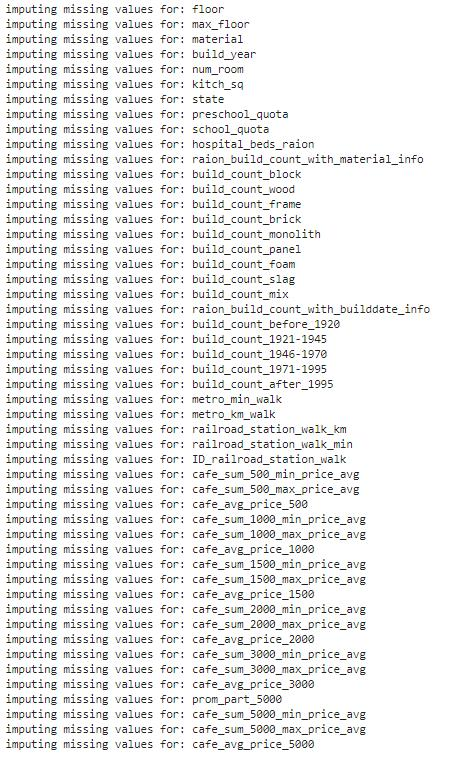# impute the missing values and create the missing value indicator variables for each non-numeric column.df_non_numeric = df.select_dtypes(exclude=[np.number])non_numeric_cols = df_non_numeric.columns.values
for col in non_numeric_cols: missing = df[col].isnull() num_missing = np.sum(missing) if num_missing > 0: # only do the imputation for the columns that have missing values. print('imputing missing values for: {}'.format(col)) df['{}_ismissing'.format(col)] = missing top = df[col].describe()['top'] # impute with the most frequent value. df[col] = df[col].fillna(top)

# categoricaldf['sub_area'] = df['sub_area'].fillna('_MISSING_')
# numericdf['life_sq'] = df['life_sq'].fillna(-999)

# histogram of life_sq.df['life_sq'].hist(bins=100)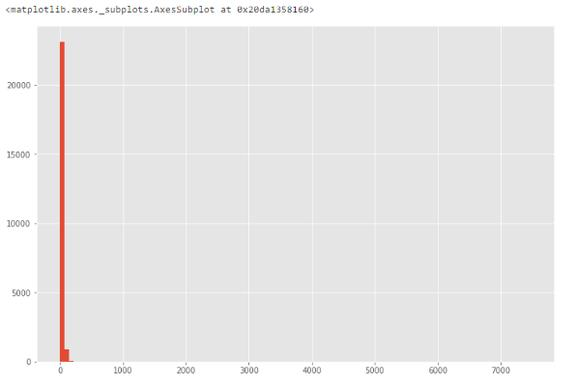# box plot.df.boxplot(column=['life_sq'])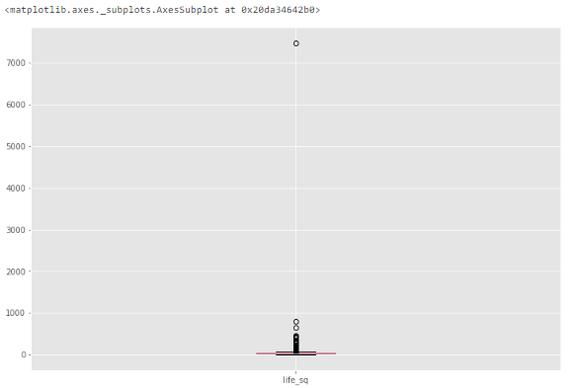df['life_sq'].describe()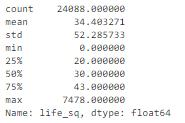# bar chart -  distribution of a categorical variabledf['ecology'].value_counts().plot.bar()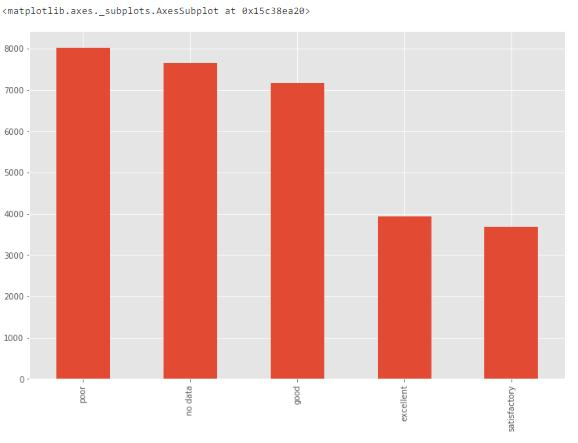num_rows = len(df.index)low_information_cols = [] #
for col in df.columns: cnts = df[col].value_counts(dropna=False) top_pct = (cnts/num_rows).iloc if top_pct > 0.95: low_information_cols.append(col) print('{0}: {1:.5f}%'.format(col, top_pct*100)) print(cnts) print()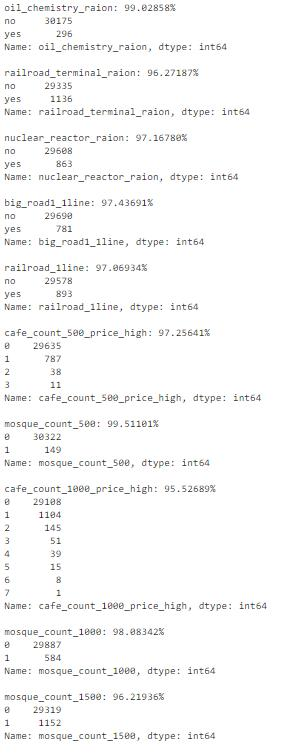# we know that column 'id' is unique, but what if we drop it?df_dedupped = df.drop('id', axis=1).drop_duplicates()
# there were duplicate rowsprint(df.shape)print(df_dedupped.shape)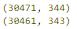key = ['timestamp', 'full_sq', 'life_sq', 'floor', 'build_year', 'num_room', 'price_doc']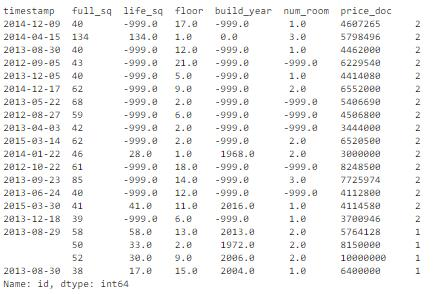# drop duplicates based on an subset of variables.
key = ['timestamp', 'full_sq', 'life_sq', 'floor', 'build_year', 'num_room', 'price_doc']df_dedupped2 = df.drop_duplicates(subset=key)
print(df.shape)print(df_dedupped2.shape)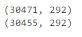### 联系我们

•### 18970825117 189709851028年专注网站建设

•### 350941543倾力打造优质产品

•### 18970985102一对一为您量身设计

•### 18970825117100+用户诚意推荐

• 商务咨询

• 联系客服

• 电话咨询

• 咨询热线 : 18970825117

• 微信互通微信扫一扫